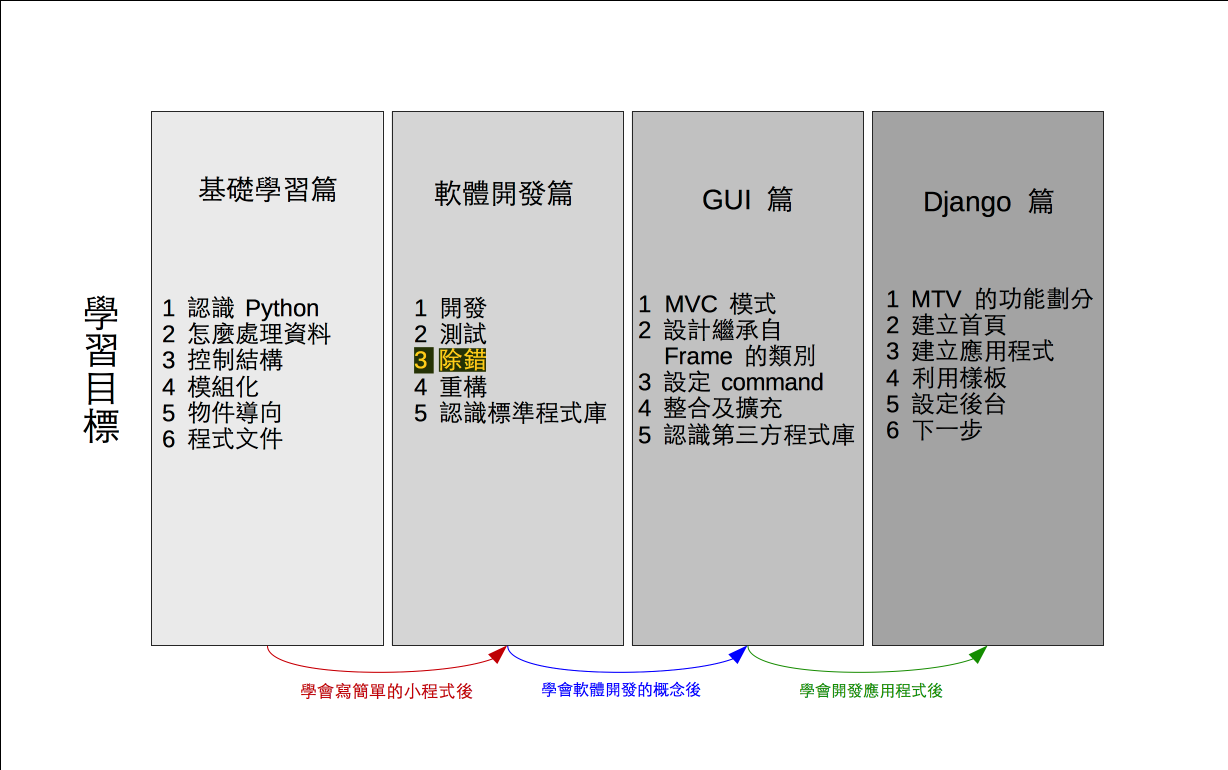# 單元 17 - 修正後的數學公式

～～學習進度表～～Syntax Errors
→ compiler or interpreter
Runtime Errors
→ exception handling
Semantic Errors
→ programmer

``````y = a * x + b
m = y % n
r = m + diff``````

``````# 使用 randint()
import random

# 定義 Encrypt 類別
class Encrypt:
def __init__(self):
self.setcode()

def setcode(self):
# 取得 a 、 b 值
a = random.randint(0, 9)
print(a) # 印出 a
b = random.randint(0, 9)
print(b) # 印出 b
# 利用公式建立密碼表
self.code = ""
c = "a"
i = 0
while i < 26:
x = c
y = ord(x) * a + b
m = y % 26
self.code += chr(m + 97)
c = chr(ord(c) + 1)
i += 1

def getcode(self):
return self.code

# 編碼的方法
def toEncode(self, str):
pass

# 解碼的方法
def toDecode(self, str):
pass

# 測試部分
if __name__ == '__main__':
e = Encrypt()
print()
print(e.getcode())
print()

#《程式語言教學誌》的範例程式
# http://kaiching.org/
# 檔名：encrypt04.py
# 功能：示範利用 Python 設計 Encrypt 類別
# 作者：張凱慶 */``````

 \$ python encrypt04.py 4 9 hlptxbfjnrvzdhlptxbfjnrvzd \$

 \$ python encrypt04.py 6 2 msyekqwciouagmsyekqwciouag \$ python encrypt04.py 9 0 pyhqzirajsbktcludmvenwfoxg \$ python encrypt04.py 0 8 iiiiiiiiiiiiiiiiiiiiiiiiii \$

``````if (a % 2) != 0 {
y = a * x + b
m = y % n
r = m + diff
}``````

``````# 使用 randint()
import random

# 定義 Encrypt 類別
class Encrypt:
def __init__(self):
self.setcode()

def setcode(self):
# 取得 a 、 b 值
a = 0
b = 0
while a % 2 == 0:
a = random.randint(0, 9)
b = random.randint(0, 9)

# 利用公式建立密碼表
self.code = ""
c = "a"
i = 0
while i < 26:
x = c
y = ord(x) * a + b
m = y % 26
self.code += chr(m + 97)
c = chr(ord(c) + 1)
i += 1

def getcode(self):
return self.code

# 編碼的方法
def toEncode(self, str):
pass

# 解碼的方法
def toDecode(self, str):
pass

# 測試部分
if __name__ == '__main__':
e = Encrypt()
print()
print(e.getcode())
print()

#《程式語言教學誌》的範例程式
# http://kaiching.org/
# 檔名：encrypt05.py
# 功能：示範利用 Python 設計 Encrypt 類別
# 作者：張凱慶 */``````

 \$ python encrypt05.py zejotydinsxchmrwbglqvafkpu \$ python encrypt05.py yzabcdefghijklmnopqrstuvwx \$ python encrypt05.py kryfmtahovcjqxelszgnubipwd \$ python encrypt05.py tuvwxyzabcdefghijklmnopqrs \$

1. 程式中可能會發生語法錯誤、執行期間錯誤或語意錯誤，編譯器會直接挑出語法錯誤，而執行期間錯誤可用例外處理機制來防範。
2. 發生語意錯誤的程式可順利執行跑出結果，可是結果不符合預期。
3. Encrypt 的數學公式經過再三的測試檢驗，發現問題出在 a0 或偶數的情況，因此要避免 a0 或偶數。

1. 什麼是語法錯誤？試舉出語法錯誤的五個例子。
2. 什麼是執行期間錯誤？想一想有哪些情況會發生執行期間錯誤？又要怎麼樣防範呢？
3. 為什麼語意錯誤最麻煩呢？
4. 我們是用什麼方式找出數學公式的錯誤呢？

1. 承接上一個單元的猜數字遊戲，將新程式寫在 exercise1701.py 中，在遊戲迴圈中用變數 times 計算使用者猜測的次數，並在遊戲結束時印出 times
2. 承上題，將新程式寫在 exercise1702.py 中，設計一個檢查使用者輸入是否有重複數字的 find_number() 函數，結果回傳布林值。